# Stock-Recruitment Graphing Questions

A reply to a user’s questions with respect to stock-recruitment function graphics.
Stock-Recruitment
ggplot2
FSA
Author

Derek H. Ogle

Published

Dec 12, 2017

Modified

Dec 18, 2022

Note

The following packages are loaded for use below. I also set the default `ggplot` theme to `theme_bw()` for a classic “black-and-white” plot (rather than the default plot with a gray background).

``````library(FSA)          # for headtail(), srStarts(), srFuns()
library(dplyr)        # for filter(), mutate(), select()
library(nlstools)     # for nlsBoot()
library(ggplot2)
theme_set(theme_bw())``````

# Introduction

A fishR user recently asked me

In the book that you published, I frequently use the stock-recruit curve code. The interface that shows both the Ricker/Beverton-Holt figure with the recruit per spawner to spawner figure (i.e., the dynamic plot for `srStarts()`) has not been working for quite some time. Additionally, I can get the recruits versus spawner plot for the Beverton-Holt or Ricker curve with confidence bounds around the curve, but how do you do the same for the recruit per spawner to spawner curve?

In this post I will answer the first question and provide a possible answer to the second questions. I will use the `PSalmonAK` data used in the book and available in `FSAdata`.1 As in the book, I remove all records with missing stock (`escapement`) or recruit (`return`) data, rescale both the `escapement` and `return` variables to be 1000s of 1000s of fish (so millions of fish), create a log of returns variable (`logret`), create “recruits per spawner” (`retperesc`) and log recruits per spawner (`logretperesc`) variables, and, for convenience, remove the `harvest` and `SST` variables.

• 1 Also documented here.

• ``````data(PSalmonAK,package="FSAdata")
pinks <- PSalmonAK |>
filter(!is.na(escapement),!is.na(return)) |>
mutate(escapement=escapement/1000,return=return/1000,
logret=log(return),
retperesc=return/escapement,logretperesc=log(retperesc)) |>
select(-harvest,-SST)
``````#R|     year escapement return   logret retperesc logretperesc
#R|  1  1960      1.418  2.446 0.894454  1.724965    0.5452066
#R|  2  1961      2.835 14.934 2.703640  5.267725    1.6615986
#R|  3  1962      1.957 10.031 2.305680  5.125703    1.6342676
#R|  28 1987      4.289 18.215 2.902245  4.246911    1.4461918
#R|  29 1988      2.892  9.461 2.247178  3.271438    1.1852298
#R|  30 1989      4.577 23.359 3.150982  5.103561    1.6299386``````

# Dynamic Plot Issue

Since Ogle (2016) was published the `dynamicPlot=` argument was removed from `srStarts()` in `FSA` because the code for that argument relied on the `tcltk` package, which I found difficult to reliably support. A similar, though more manual, approach is accomplished with the new `fixed=` and `plot=` arguments. For example, using `plot=TRUE` (without `fixed=`) generates a plot of “recruits” versus “stock” with the chosen stock-recruitment model evaluated at the automatically chosen parameter starting values superimposed.

``svR <- srStarts(return~escapement,data=pinks,type="Ricker",plot=TRUE)``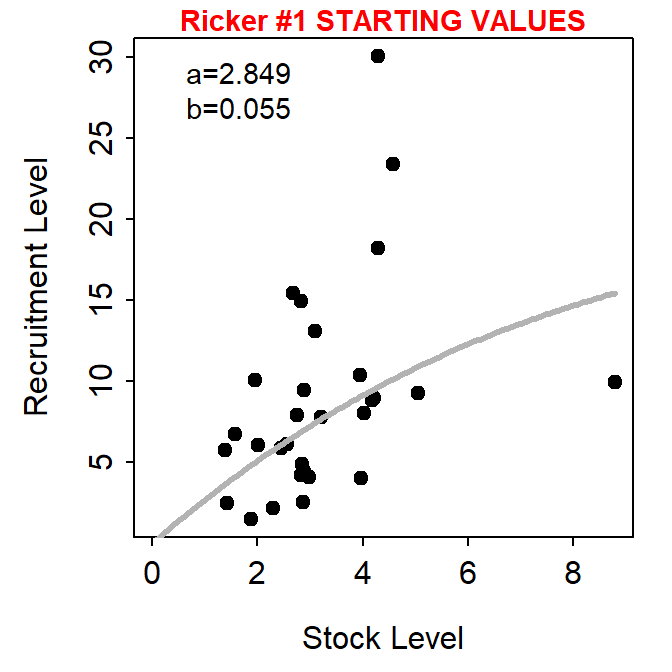Figure 1: Recruitment versus stock plot with the Ricker function evaluated at the automatically chosen starting values superimposed.

The user, however, can show the stock-recruitment model evaluated at manually chosen parameter starting values by including those starting values in a named `list()` supplied to `fixed=`. These values can be iteratively changed in subsequent calls to `srStarts()` to manually find starting values that provide a model that reasonably fits (by eye) the stock-recruit data.

``````svR <- srStarts(return~escapement,data=pinks,type="Ricker",plot=TRUE,
fixed=list(a=4,b=0.15))``````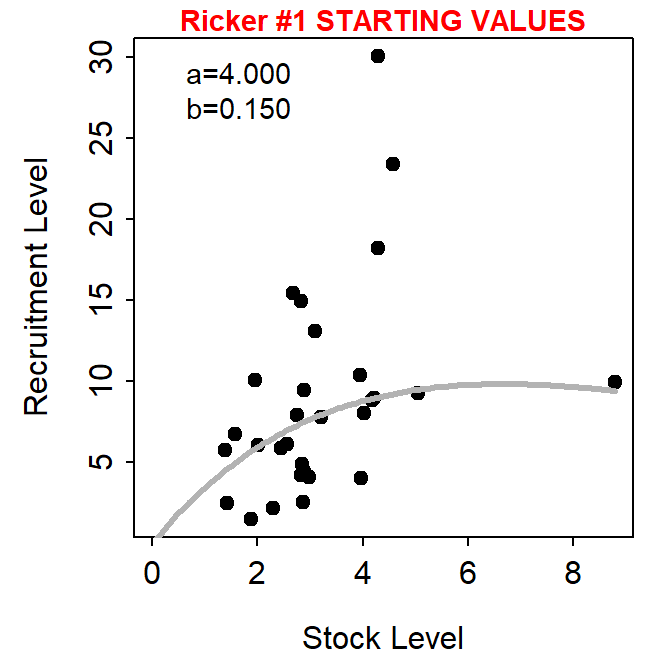Figure 2: Recruitment versus stock plot with the Ricker function evaluated at the manually chosen starting values superimposed.
Important

`srStarts()` no longer, however, supports the simultaneously plotting of spawners versus recruits and recruits per spawner versus recruits.

# Plot of Recruits per Spawner versus Spawners

The first way that I imagined plotting recruits per spawners versus spawners with the fitted curve and confidence bands is to first follow the code for fitting the stock-recruit function to the stock and recruit data as described in Ogle (2016). In this case, the stock-recruit function is fit on the log scale to adjust for a multiplicative error structure (as described in the book).2

• 2 The manually selected starting values from above are used here.

• ``````rckr <- srFuns("Ricker")
srR <- nls(logret~log(rckr(escapement,a,b)),data=pinks,start=svR)
bootR <- nlsBoot(srR)
cbind(estimates=coef(srR),confint(bootR))``````
``````#R|     estimates     95% LCI   95% UCI
#R|  a 2.84924199  1.70935506 4.6711392
#R|  b 0.05516673 -0.08391462 0.1994054``````

Ogle (2016) showed how to plot spawners versus recruits using base graphics. Here however I will use `ggplot2`. Either method first requires (i) constructing a sequence of “x” values that span the range of observed numbers of spawners,3 (ii) predicting the number of recruits at each spawner value using the best-fit stock-recruitment model, and (iii) constructing lower and upper confidence bounds for the predicted number of recruits at each spawner value with the bootstrap results. These results are assigned to a data framed called `preds` below.

• 3 Increase the value in `length.out=` for a smoother curve and band.

• ``````x <- seq(0,9,length.out=199)        # many S for prediction
pR <- rckr(x,a=coef(srR))           # predicted mean R
LCI <- UCI <- numeric(length(x))

for(i in 1:length(x)) {             # CIs for mean R @ each S
tmp <- apply(bootR\$coefboot,MARGIN=1,FUN=rckr,S=x[i])
LCI[i] <- quantile(tmp,0.025)
UCI[i] <- quantile(tmp,0.975)
}
preds <- data.frame(escapement=x,return=pR,LCI=LCI,UCI=UCI)
``````#R|      escapement     return        LCI        UCI
#R|  1   0.00000000  0.0000000 0.00000000  0.0000000
#R|  2   0.04545455  0.1291866 0.07794713  0.2103041
#R|  3   0.09090909  0.2577262 0.15650236  0.4166059
#R|  197 8.90909091 15.5279219 6.50772063 34.9827333
#R|  198 8.95454545 15.5680589 6.48296004 35.2738317
#R|  199 9.00000000 15.6078974 6.45812729 35.5664363``````

The recruits versus spawners graph is then constructed in `ggplot2` by adding the 95% confidence band from `preds` using `geom_ribbon()`, adding the best-fit model curve from `preds` using `geom_line()`, and adding the observed data from `pinks` using `geom_point()`.4

• 4 I plot the layers in this order so that the line is on top of the confidence band and the points are on top of both the line and band.

• ``````ggplot() +
geom_ribbon(data=preds,mapping=aes(x=escapement,ymin=LCI,ymax=UCI),
fill="gray50",alpha=0.5) +
geom_line(data=preds,mapping=aes(x=escapement,y=return),linewidth=1) +
geom_point(data=pinks,mapping=aes(x=escapement,y=return)) +
scale_x_continuous(name="Escapement (millions)") +
scale_y_continuous(name="Returners (millions)")``````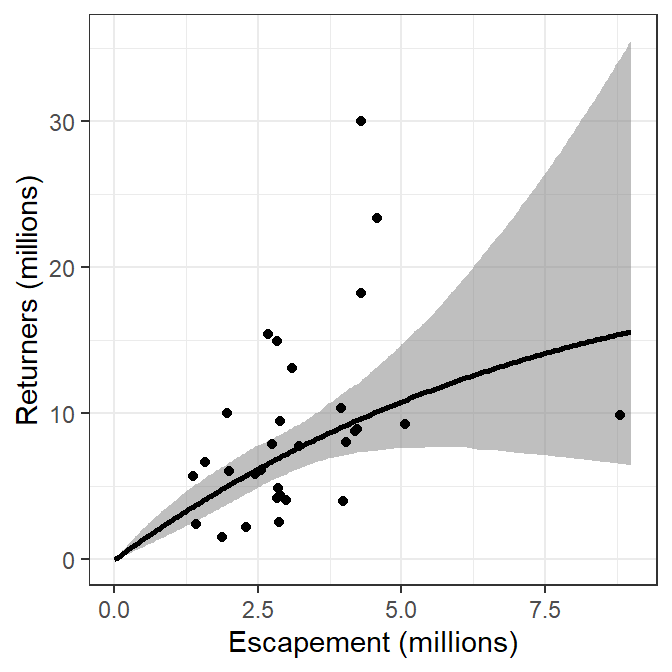Figure 3: Returners versus escapement for Pacific Salmon with the best-fit Ricker recruitment function and bootstrapped 95% confidence band.

These results can be modified to plot recruits per spawner versus spawners by replacing the “recruits” in the code above with “recruits per spawner.” This is simple for the observed data as `return` is simply replaced with `retperesc`. However, the predicted number of recruits (`return` in `preds`) and the confidence bounds (`LCI` and `UCI` in `preds`) from above must be divided by the number of spawners (`escapement` in `preds`). The `preds` data frame is modified accordingly below.

``````preds <- preds |>
mutate(retperesc=return/escapement,
rpeLCI=LCI/escapement,
rpeUCI=UCI/escapement)``````

The plot is then constructed with the appropriate modification of variable names and axis labels.5

• 5 I used red for the confidence band here for illustrative purposes below, usually I would use a gray as in Figure 3.

• ``````ggplot() +
geom_ribbon(data=preds,mapping=aes(x=escapement,ymin=rpeLCI,ymax=rpeUCI),
fill="red",alpha=0.5) +
geom_line(data=preds,mapping=aes(x=escapement,y=retperesc),linewidth=1) +
geom_point(data=pinks,mapping=aes(x=escapement,y=retperesc)) +
scale_x_continuous(name="Escapement (millions)") +
scale_y_continuous(name="Returners/Escapement")``````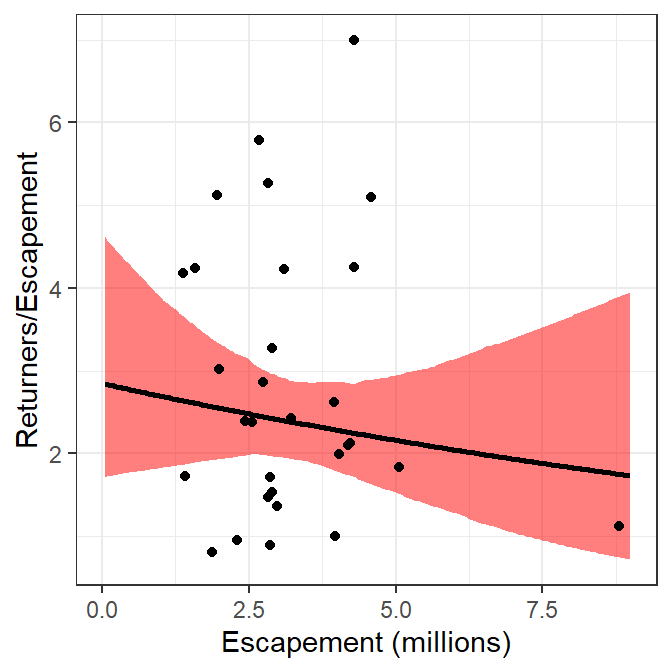Figure 4: Returners per escapement versus escapement for Pacific Salmon with the best-fit Ricker recruitment function and bootstrapped 95% confidence band.

Alternatively, the Ricker model could be reparameterized by dividing each side of the function by “spawners” such that the left-hand-side becomes “recruits per spawner.”6 This recruitment model can be put into an R function, with parameters estimated with nonlinear regression similar to above. The results below show that the parameter point estimates are identical and the bootsrapped confidence intervals are similar to what was obtained above.

• 6 This is a fairly typical reparameterization of the Ricker model.

• ``````rckr2 <- function(S,a,b=NULL) {
if (length(a)>1) { b <- a[]; a <- a[] }
a*exp(-b*S)
}
srR2 <- nls(logretperesc~log(rckr2(escapement,a,b)),data=pinks,start=svR)
bootR2 <- nlsBoot(srR2)
cbind(estimates=coef(srR2),confint(bootR2))``````
``````#R|     estimates     95% LCI   95% UCI
#R|  a 2.84924192  1.67775104 4.9025369
#R|  b 0.05516672 -0.08979965 0.2103898``````

With this, a second method for plotting recruits per spawner versus spawners is the same as how the main plot from the book was constructed but modified to use the results from this reparameterized function.

``````x <- seq(0,9,length.out=199)        # many S for prediction
pRperS <- rckr2(x,a=coef(srR2))     # predicted mean RperS
LCI2 <- UCI2 <- numeric(length(x))

for(i in 1:length(x)) {             # CIs for mean RperS @ each S
tmp <- apply(bootR2\$coefboot,MARGIN=1,FUN=rckr2,S=x[i])
LCI2[i] <- quantile(tmp,0.025)
UCI2[i] <- quantile(tmp,0.975)
}
preds2 <- data.frame(escapement=x,retperesc=pRperS,rpeLCI=LCI2,rpeUCI=UCI2)
``````#R|      escapement retperesc    rpeLCI   rpeUCI
#R|  1   0.00000000  2.849242 1.6777510 4.902537
#R|  2   0.04545455  2.842106 1.6841248 4.846330
#R|  3   0.09090909  2.834988 1.6905230 4.796657
#R|  197 8.90909091  1.742930 0.6884826 4.213108
#R|  198 8.95454545  1.738565 0.6821556 4.229946
#R|  199 9.00000000  1.734211 0.6758867 4.246851``````
``````ggplot() +
geom_ribbon(data=preds2,mapping=aes(x=escapement,ymin=rpeLCI,ymax=rpeUCI),
fill="blue",alpha=0.5) +
geom_line(data=preds2,mapping=aes(x=escapement,y=retperesc),linewidth=1) +
geom_point(data=pinks,mapping=aes(x=escapement,y=retperesc)) +
scale_x_continuous(name="Escapement (millions)") +
scale_y_continuous(name="Returners/Escapement")``````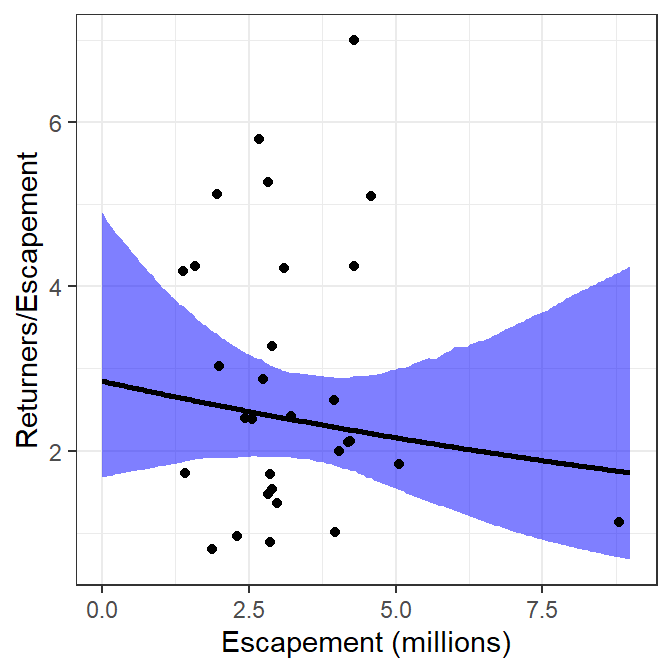Figure 5: Returners per escapement versus escapement for Pacific Salmon with the best-fit Ricker recruitment function and bootstrapped 95% confidence band.

The two methods described above for plotting recruits per spawner versuse spawners are identical for the best-fit curve and nearly identical for the confidence bounds (slight differences likely due to the randomness inherent in bootstrapping). Thus, the two methods produce nearly the same visual.

``````ggplot() +
geom_ribbon(data=preds,mapping=aes(x=escapement,ymin=rpeLCI,ymax=rpeUCI),
fill="red",alpha=0.25) +
geom_ribbon(data=preds2,mapping=aes(x=escapement,ymin=rpeLCI,ymax=rpeUCI),
fill="blue",alpha=0.25) +
geom_line(data=preds,mapping=aes(x=escapement,y=retperesc),linewidth=1) +
geom_line(data=preds2,mapping=aes(x=escapement,y=retperesc),linewidth=1) +
geom_point(data=pinks,mapping=aes(x=escapement,y=retperesc)) +
scale_x_continuous(name="Escapement (millions)") +
scale_y_continuous(name="Returners/Escapement")``````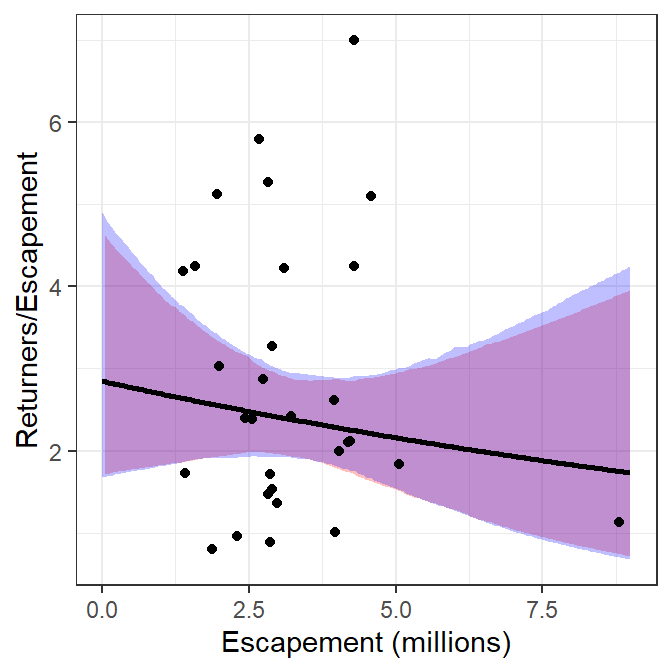Figure 6: Returners per escapement versus escapement for Pacific Salmon with the best-fit Ricker recruitment function and bootstrapped 95% confidence band shown for both estimation methods.

## References

Ogle, D. H. 2016. Introductory Fisheries Analyses with R. CRC Press, Boca Raton, FL.

## Citation

BibTeX citation:
``````@online{h.ogle2017,
author = {Derek H. Ogle},
title = {Stock-Recruitment {Graphing} {Questions}},
date = {2017-12-12},
url = {https://fishr-core-team.github.io/fishR//blog/posts/2017-12-12_StockRecruit_Graph_Questions},
langid = {en}
}
``````